### Variations on the Buddhabrot

• 8 Replies
• 711 Views

0 Members and 1 Guest are viewing this topic.

####AlexH#### Variations on the Buddhabrot

« on: September 08, 2018, 10:54:32 PM »
I've had a number of ideas over the past couple months regarding variations on the Buddhabrot.
I don't have a ton of time today to go over the finer points of  exactly how each of these work, but they all relate to a common concept: What does it look like as each complex point moves linearly between each of its iterations?

Naming:

If this is in fact a new idea I kind've like the term "Projectilebrot", with each of my current examples being under the sub-category of "Linear Projectilebrot".
Other ideas:
"Particlebrot" (I really like this one, but feel like it should be reserved for something else.)
"Trajectorybrot" (Unweildy.)
"Laserbrot" (Only works if the movement is linear, and although all of my implementations are linear, they don't have to be.)

For the time being, all implementations that involve drawing lines will be called "Laserbrots", and animations showing step-by-step movement are called "Particlebrots".

Explanations:

Particlebrot (animation-exclusive): Move all points ([frame #]/[#of frames])% of the way to their destination each frame.
I have evidence that this isn't an entirely original idea. I recently found someone who had done something like this before, but they used non-linear movement.
https://www.youtube.com/watch?v=yH9QZ_c3R3Q

Laserbrot: For each iteration, imagine drawing a line between $$Z_{n}$$ and $$Z_n+1$$. For each bucket the line passes through, calculate the distance the line travels through that particular bucket and add that distance to that bucket.

Riemann Laserbrot: Construct a sphere from voxels. The voxels will act as our buckets. Convert your $$Z_{n}$$ and $$Z_n+1$$ coordinates to coordinates on the Riemann sphere. Imagine drawing a line between each point DIRECTLY THROUGH the sphere. For each voxel-bucket the line passes through, calculate the distance the line travels through that particular voxel-bucket and add that distance to that voxel-bucket.

Examples:

Note All of my implementations are still a little buggy. Also, I only very recently added the ability for my program to distinguish between Normal and Anti-Buddhabrots, so most of these examples are Normal+Anti.

(Attached) Laserbrot (Normal + Anti) - Kinda looks like Buddha's rib cage.

Particlebrot (Normal + Anti) - This is one I created early on. I have since made a change to the algorithm that fades in the first iteration and fades out the last one, which makes the animation loop smoother. There's still a bug with it, so it doesn't work as intended all the time.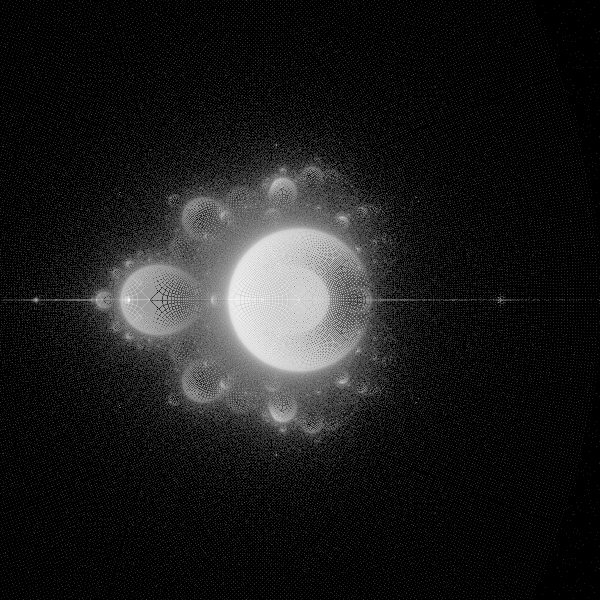Riemann Anti-Laserbrot - I just completed this last night. This animation cycles through the XY, ZY, and ZX cross-sections of the sphere. The next thing I want to do is render the whole thing in 3D (See Future Ideas).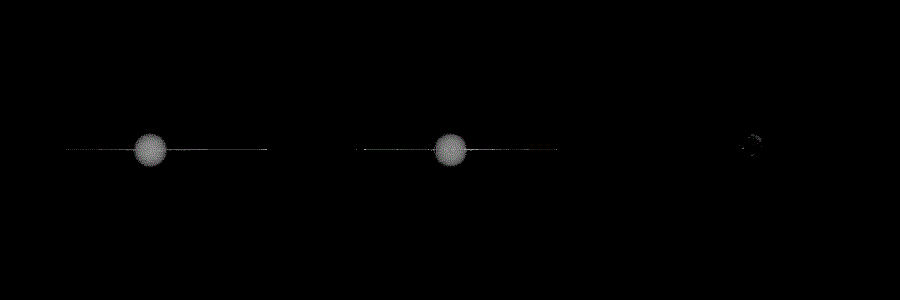Other Examples:

Particlebrot (Not a Mandelbrot, will add equation later.) (Normal + Anti) - This is my favorite Particlebrot.
https://media.giphy.com/media/oNTKF9FfzGJOXxrRH0/giphy.gif

Laserbrot Animation (Not a Mandelbrot, will add equation later.) (Normal + Anti) - Cycling through a series of Julias.
https://media.giphy.com/media/1lyN7GySu1FwP6nDs6/giphy.gif

Future ideas not yet implemented:

The Riemann Laserbrot is incomplete. I want to render it in 3D, but on top of that I have a few ideas regarding some rendering effects.
1. Normal render.
2. Value-based opacity. All voxels are white, but their opacity is based on their value.
3. Perspective-based brightness. Recompute the brightness of each pixel based on the angle you're looking at the sphere, adding the obscured voxels values to the values of the voxels in front of them.

I'd also like to do the same thing with plain-old Buddhabrots on the Riemann Sphere. Also, I'd like to make an iteration-layer based version of the Buddhabrot on the Riemann Sphere, just like I've done before with fractals:
https://media.giphy.com/media/xUA7b2tiISfH8Jw47K/giphy.gif

I'd also like to explore more non-linear movements. After all, $${Z_{n}}^2+C$$ is a rotation and then a translation (or vice-versa). Showing how those two ideas work together could also result in something cool.

Final note:

I wrote this all in a 2.5-hour rush so I know I missed something or could have explained something better (Like why would fading in the first and fading out the last iteration on a Particlebrot make an animation loop more smoothly?). I also want to add some more examples that I either don't have rendered or don't have good renders of.
« Last Edit: March 03, 2019, 09:00:16 PM by AlexH »

####claude#### Re: (New?) Variations On The Buddhabrot

« Reply #1 on: September 18, 2018, 12:26:38 PM »
Quote
Particlebrot (Normal + Anti)
Great animation ideaYou can really see the rotation of the bulbs around rational parabolic points.

For the Anti (which is my preferred variant), you could colour based on period of the limit cycle, which might bring out more structure to the eye.  I have some code for such an Anti-Buddhabrot here: https://mathr.co.uk/blog/2013-12-30_ultimate_anti-buddhabrot.html (the "ultimate" is just because it finds the limit cycle, instead of plotting all iterates, not because it's the best way of doing it).  My code only plots periodic interior points, though - I'm not sure if the other boundary points (Misiurewicz, Siegel, etc) would contribute anything visible, it depends on whether the boundary of the Mandelbrot set (which has dimension 2) has a positive area, I think that question is still an open research problem...

It could also be fun to interpolate the periodic cycle using cubic splines instead of linear segments, which should give a less steppy appearance.  I wonder what the most mathematically meaningful way of doing it would be.  Maybe:
$w_k(t) = z_k^{1 + t} + t c$
but I think you'd want to pick a continuous branch for the power to minimize the total arc length of $$w_k(t), t \in [0,1)$$, and I'm not sure how to go about that - perhaps for power 2 it's enough to consider t = 1/2 and pick the w that is nearest the straight line midpoint (z + z^2+c)/2 ?

####AlexH#### Re: (New?) Variations On The Buddhabrot

« Reply #2 on: September 20, 2018, 03:51:25 AM »
Wait, you're the person who created that image? I found your article through google last year while I was researching how Buddhabrots were colored. Not a joke: that's my absolute favorite Anti-Buddhabrot. So actually, yeah, I think "Ultimate" is pretty appropriate. XD
I never noticed the source code at the bottom of the page. I'll definitely look into it.

Embarrassingly, I don't know that much about the properties of the Mandelbrot Set. I tend to stray away from Mandelbrot-related stuff except when I'm testing new ideas out. I don't hate the Mandelbrot Set or anything, it's just that my favorite part of generating fractals is mixing different equations and escape rules together and seeing what pops out.

Anyway, the point is that you threw like, 6 vocabulary terms at me and I had no idea what they meant. XD
I'm thrilled to have an excuse to finally learn some of the stuff that I'd been putting off learning for so long.

I understand your equation though. I'll give it a shot and post the results soon.

If there is a mathematically-significant way of moving a point across the plane, then I would guess that there would be movement rules for the building blocks of mathematics that would remain the same even if you rewrote the equation and used identities. For instance, the movement for $$ln({Z_{n}}^{2})$$ would be the same as the movement for $$ln(Z_{n}) + ln(Z_{n})$$. Intuitively I think that addition is probably linear movement, but intuition isn't always reality.

Other stuff:

Here's a higher-quality Normal and Anti Particlebrot: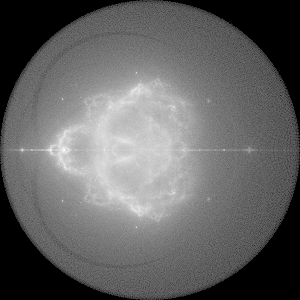Here's a Normal Julia Set Particlebrot for [latex=inline]C=0+0*i[/inline]: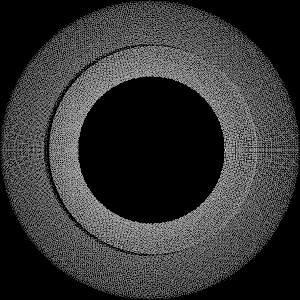Here's one thing I forgot to show off last time. If you gradually change the C value of a Julia Set Particlebrot every frame, as long as you return C to its initial value at the end of the animation you can create a loop. This is probably obvious for some but I thought it was worth sharing.
https://media.giphy.com/media/XKJ53oEvXPFd49Tia4/giphy.gif

####AlexH#### Re: (New?) Variations On The Buddhabrot

« Reply #3 on: September 22, 2018, 10:39:06 PM »
Status report:
This morning I attempted to implement the new movement suggested by Claude and was met with a bug: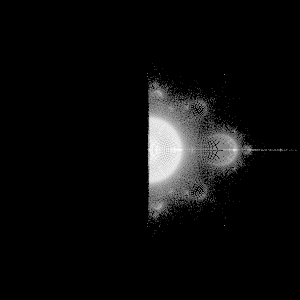Apparently there's a major bug in my generator where the Complex.pow(Complex input) function does not return the correct output. This is a pretty horrifying revelation for me because some of my favorite fractals I've made (and at least one or two I've posted to the gallery) use that function.

I attempted to fix it, but was met with further problems. I did manage to make one that was pretty cool, but it was not what Claude suggested.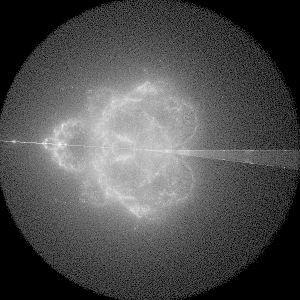Tomorrow I will attempt again.

####claude#### Re: (New?) Variations On The Buddhabrot

« Reply #4 on: September 23, 2018, 02:31:30 PM »
I attempted to fix it

Keep a backup of the old version to be able to reproduce old images!

####AlexH#### Re: (New?) Variations On The Buddhabrot

« Reply #5 on: September 24, 2018, 03:48:54 AM »
Keep a backup of the old version to be able to reproduce old images!
Haha, absolutely.
There was something wrong with my arg function, which is used by my pow function.

Here's the results: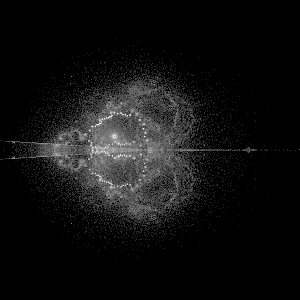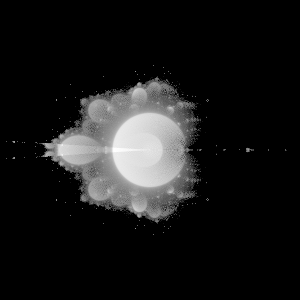This is the same movement that can be seen in the youtube video by Ahknaton I linked to in my first post.
https://www.youtube.com/watch?v=yH9QZ_c3R3Q

####AlexH#### Re: (New?) Variations On The Buddhabrot

« Reply #6 on: February 03, 2019, 07:24:53 PM »
I've been meaning to add this for a while now. This is the 3D version of the Cross-Sections seen in the first post. It's still a bit buggy,Explanation:
The surface of the sphere is a Riemann Sphere. The sphere is made of voxels that act like buckets in a traditional 2D Buddhabrot. Each iteration, a straight line is drawn through the sphere from $$Z_n$$ to $$Z_{n+1}$$. All of the voxel-buckets that the line passes through have a value added to them. That value is the length of the section of the line that passes through that voxel. The voxels are 1x1x1 cubes, so the maximum value that can be added to the bucket on any given iteration is $$\frac{\sqrt[]{3}}{2}$$ (that's if the line passes directly through a diagonal).

The final image is made by creating a traditional 2D array of buckets, rotating the sphere to the desired angle, then adding up the values from all of the voxels that are on top of each other at that angle. (I think the reason why my animation flickers every 30 frames is that the particular angle the voxels are being viewed from are causing them to be aligned in an unfavorable way.. I'm not truly rendering voxels yet. I'm just treating them like points in a 3D array.) My version doesn't currently have any 3D perspective to it, so it's really easy to get confused as to which way the sphere is facing.

Cross-Sections:I attempted a solid version a while back, but it was awful. What I really need to make it work is an actual voxel renderer with a light+shadow engine, but I also need to rethink the way it's being colored. Also, this wasn't clear in the transparent version, but even the black spaces have values in them. I had to use a threshold to exclude smaller values because otherwise this version would look like a solid sphere.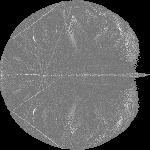####AlexH#### Re: (New?) Variations On The Buddhabrot

« Reply #7 on: February 09, 2019, 06:37:41 AM »
Apologies for triple-posting. I'm not quite sure whether it's appropriate make a new post if you've done something new, or if you should just update your last post. If this isn't appropriate let me know.

I tried applying some color to my renders by compositing multiple spheres and I thought the results were pretty cool. For all three renders, the parameters for the spheres were:
Red = 300 iterations *edit* 100 iterations
Green = 30 iterations
Blue = 3 iterations

Cross Sections:Before the frames are created, the max value is found inside the the three spheres. The output RGB values are generated relative to the max value found in their respective spheres.

Transparent Sphere 1: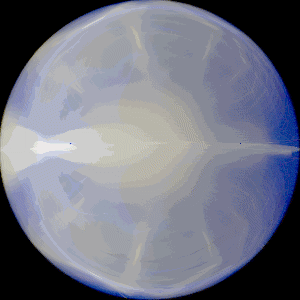(Sadly, the .gif format's limited color capabilities really did a number on this one, so I have attached some of the still renders below.)
The Color is calculated on a frame-by-frame basis after totaling the points that overlap in the sphere at the current angle and finding the maximum value. Honestly, the color is a lot more stable at all angles than I thought it would be. I guess that makes sense since the black and white version was also pretty stable. There's a tiny bit of pearlescence (*edit* Actually I think pearlescence is based on the way light hits a surface, which has nothing to do with this. It just happens to look pearl-esque.) there which is kind've cool, but I was hoping for something a little more dramatic.

Transparent Sphere 2: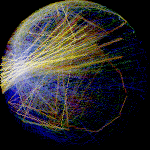This sphere is exactly the same as the one above, except it has fewer input values (also it's spinning differently, but that's not what makes it look so different). The sphere above used a 900x900 grid of $$Z_0$$ values, with a domain and range of $$[-2,2]$$. The one below still has a domain and range of $$[-2,2]$$, but it only uses a 45x45 grid of $$Z_0$$ values.
The optical illusion is pretty strong in this one. For the record, it's spinning clockwise, but you'd be forgiven for thinking otherwise.
« Last Edit: February 09, 2019, 07:10:22 PM by AlexH, Reason: Accidentally typed "300 iterations" instead of "100 iterations" for the red channel. »

####AlexH#### Re: (New?) Variations On The Buddhabrot

« Reply #8 on: February 11, 2019, 12:01:03 PM »
I finally got around to cleaning my up my code a bit so I could share it. The examples are all in C#, but with the exception of the Animated GIF library I used it should be extremely simple to port into any other language.

I'm pretty sure that providing any code for the Complex class is overkill, but here's an abridged version anyway:
Code: ("Complex Class") [Select]
public class Complex{ public double real; public double imag; public Complex() { this.real = 0; this.imag = 0; } public Complex(double real, double imag) { this.real = real; this.imag = imag; } public void SetValue(double real, double imag) { this.real = real; this.imag = imag; }}
The Voxel struct is extremely simple. It's basically just a float that acts as our bucket and a bool value that flags whether or not the bucket counted when rendering.
Code: ("Voxel Struct") [Select]
public struct Voxel{ public bool active; public float value; public Voxel(bool active) { this.active = active; this.value = 0; }}
Here we init all of the Voxels and carve the sphere out of a 3D array. The diameter should be equal to the width and height of the image you want to render. Setting the "active" bool to true tells the program whether or not the Voxel is part of the sphere. This serves two functions:
1. We can save some time during rendering by ignoring the "false" voxels. (in retrospect we could also check for "null" if we don't initialize them. I'm not sure which is faster in C#.)
2. The algorithm that fills the buckets is still a little buggy and will draw outside the sphere. We can clean it up by disabling rendering on all points outside of the sphere.
Code: ("Init Voxel Sphere") [Select]
public Voxel[,,] CreateVoxelSphere(int diameter){ Voxel[,,] voxelSphere = new Voxel[diameter, diameter, diameter]; //Diameter = 300 //  0 -> -150 //299 ->  150 //c = im - 150 //300 = 299m - 150 //(300 - 150) / (300 - 1) float radius = (float)diameter / 2; float m = diameter / (diameter - 1); //Calculate the coordinates of the voxel. for (int x = 0; x < diameter; x++) { float xm = (x * m) - radius; for (int y = 0; y < diameter; y++) { float ym = (y * m) - radius; for (int z = 0; z < diameter; z++) { float zm = (z * m) - radius; float distance = (float)Math.Sqrt((xm * xm) + (ym * ym) + (zm * zm)); if (distance <= radius) { voxelSphere[x, y, z] = new Voxel(true); } else { voxelSphere[x, y, z] = new Voxel(false); } //Console.WriteLine(xm + ", " + ym + ", " + zm); if (z == 0 && y == 0) { Console.WriteLine(x + ", " + y + ", " + z); } } } } return voxelSphere;}
Point3D is a struct that is part of System.Windows.Media.Media3D, but all I use it for is to store three doubles that represent 3D space.
Code: ("Point3D Struct") [Select]
public struct Point3D{ public double X; public double Y; public double Z; public Point3D() { this.X = 0; this.Y = 0; this.Z = 0; } public Point3D(double x, double y, double z) { this.X = x; this.Y = y; this.Z = z; }}
This converts a Complex number into a 3D point on the sphere.
Code: ("Complex to Riemann Sphere") [Select]
static Point3D ConvertComplexToRiemannCoordinates(Complex input){ double realSquare = input.real * input.real; double imagSquare = input.imag * input.imag; double x = (2 * input.real) / (1 + realSquare + imagSquare); double y = (2 * input.imag) / (1 + realSquare + imagSquare); double z = (realSquare + imagSquare - 1) / (1 + realSquare + imagSquare); return new Point3D(x, y, z);}
This is the slightly buggy algorithm that will fill the sphere. At the end of each iteration in your fractal generator, convert $$Z_n$$ and $$Z_{n-1}$$ into Point3D's using the above function and send them to this function along with the Voxel Sphere.
Code: ("Algorithm") [Select]
static void RiemannVoxelTravelAlgorithm(Point3D start, Point3D end, Voxel[,,] voxelSphere){ //Rise Over Run double xyRateOfChange = (end.Y - start.Y) / (end.X - start.X); //b = y - mx double xy_yIntercept = start.Y - (xyRateOfChange * start.X); double yzRateOfChange = (end.Z - start.Z) / (end.Y - start.Y); double yz_zIntercept = start.Z - (yzRateOfChange * start.Y); int directionX; int directionY; int directionZ; int nextIntX; int nextIntY; int nextIntZ; if (start.X < end.X) { directionX = 1; nextIntX = (int)Math.Ceiling(start.X); } else { directionX = -1; nextIntX = (int)Math.Floor(start.X); } if (start.Y < end.Y) { directionY = 1; } else { directionY = -1; } if (start.Z < end.Z) { directionZ = 1; } else { directionZ = -1; } Point3D currentSubX = new Point3D(start.X, start.Y, start.Z); Point3D nextSubX = new Point3D(end.X, end.Y, end.Z); Point3D currentSubY = new Point3D(); Point3D nextSubY = new Point3D(); Point3D currentSubZ = new Point3D(); Point3D nextSubZ = new Point3D(); int numberOfXSteps = (int)Math.Abs(Math.Floor(start.X) - Math.Floor(end.X)) + 1; Console.Write(numberOfXSteps + " "); for (int x = 1; x <= numberOfXSteps && x < voxelSphere.GetLength(0); x++) { //Is this the last step? if (x != numberOfXSteps) { //If not, calculate the next point. //Solve for y at the given x. //y = mx + b double subX_y = (xyRateOfChange * nextIntX) + xy_yIntercept; //Solve for z at the given y. //z = my + b double subX_z = (yzRateOfChange * subX_y) + yz_zIntercept; nextSubX.X = nextIntX; nextSubX.Y = subX_y; nextSubX.Z = subX_z; } else { //Otherwise, set the next point to the value of the end point. nextSubY.X = end.X; nextSubY.Y = end.Y; nextSubY.Z = end.Z; } //Calculate the number of Y sub-steps. //A sub-step occurs when the line passes an integer threshold on the y-axis. int numberOfYSubSteps = (int)Math.Abs(Math.Floor(currentSubX.Y) - Math.Floor(nextSubX.Y)) + 1; currentSubY = new Point3D(currentSubX.X, currentSubX.Y, currentSubX.Z); int currentIntY; if (start.Y < end.Y) { currentIntY = (int)Math.Ceiling(currentSubY.Y); } else { currentIntY = (int)Math.Floor(currentSubY.Y); } nextIntY = currentIntY - directionY; for (int y = 1; y <= numberOfYSubSteps; y++) { if (y != numberOfYSubSteps) { nextIntY += directionY; nextSubY.Y = nextIntY; //Calculate the next sub-point. //Solve for x at the given y. //y = mx + b //y - b = mx //(y - b) / m = x //x = (y - b) / m nextSubY.X = (nextIntY - xy_yIntercept) / xyRateOfChange; // Solve for z at the given y. //z = my + b nextSubY.Z = (yzRateOfChange * nextSubY.Y) + yz_zIntercept; } else { //Otherwise, set the next point to the value of the end point. nextSubY.X = nextSubX.X; nextSubY.Y = nextSubX.Y; nextSubY.Z = nextSubX.Z; } //Calculate the number of Y sub-steps. //A sub-step occurs when the line passes an integer threshold on the y-axis. int numberOfZSubSteps = (int)Math.Abs(Math.Floor(currentSubY.Z) - Math.Floor(nextSubY.Z)) + 1; currentSubZ = new Point3D(currentSubY.X, currentSubY.Y, currentSubY.Z); int currentIntZ; if (start.Z < end.Z) { currentIntZ = (int)Math.Ceiling(currentSubZ.Z); } else { currentIntZ = (int)Math.Floor(currentSubZ.Z); } nextIntZ = currentIntZ - directionZ; for (int z = 1; z <= numberOfZSubSteps; z++) { if (z != numberOfZSubSteps) { nextIntZ += directionZ; nextSubZ.Z = nextIntZ; //Calculate the next sub point. //yz //Solve for y at the given z. //z = my + b //z - b = my //(z - b) / m = y //y = (z - b) / m nextSubZ.Y = (nextIntZ - yz_zIntercept) / yzRateOfChange; //Solve for x at the current y. //y = mx + b //y - b = mx //(y - b) / m = x //x = (y - b) / m nextSubZ.X = (nextSubZ.Y - xy_yIntercept) / xyRateOfChange; } else { //Otherwise, set the next point to the value of the end point. nextSubZ.X = nextSubY.X; nextSubZ.Y = nextSubY.Y; nextSubZ.Z = nextSubY.Z; } int bucketX = (int)Math.Floor(nextSubZ.X); int bucketY = (int)Math.Floor(nextSubZ.Y); int bucketZ = (int)Math.Floor(nextSubZ.Z); if (bucketX >= 0 && bucketX < voxelSphere.GetLength(0) && bucketY >= 0 && bucketY < voxelSphere.GetLength(1) && bucketZ >= 0 && bucketZ < voxelSphere.GetLength(2)) { //Perform heat map operations here. //Calculate the distance between currentSub and nextSub. double deltaX = currentSubZ.X - nextSubZ.X; double deltaY = currentSubZ.Y - nextSubZ.Y; double deltaZ = currentSubZ.Z - nextSubZ.Z; double delta = Math.Sqrt((deltaX * deltaX) + (deltaY * deltaY) + (deltaZ * deltaZ)); voxelSphere[bucketX, bucketY, bucketZ].value += (float)(delta / root3); } else { //For Debugging //Console.WriteLine(bucketX + ", " + bucketY + ", " + bucketZ); } currentSubZ.X = nextSubZ.X; currentSubZ.Y = nextSubZ.Y; currentSubZ.Z = nextSubZ.Z; } currentSubY.X = nextSubY.X; currentSubY.Y = nextSubY.Y; currentSubY.Z = nextSubY.Z; } currentSubX.X = nextSubX.X; currentSubX.Y = nextSubX.Y; currentSubX.Z = nextSubX.Z; nextIntX += directionX; }}
This is a really simple function that just finds the maximum value in the given array. It's used in the rendering method that's coming up next.
Code: ("Find Max Double") [Select]
private double FindMaxDoubleIn2DArray(double[,] doubleArray){ double maxDouble = double.MinValue; for (int x = 0; x < doubleArray.GetLength(0); x++) { for (int y = 0; y < doubleArray.GetLength(1); y++) { if (doubleArray[x, y] > maxDouble) { maxDouble = doubleArray[x, y]; } } } return maxDouble;}
This is the code for the RGB version of animation renderer. Send it three spheres, each generated from the same function with different maximum iterations.
In this code Bitmap is a C# image class and AnimatedGIF is an external library I used. If you're porting this to another language you'll need to find replacements.
Code: ("Rendering") [Select]
static void VoxelSphereAnimationRGB(string folder, string fractalName, Voxel[,,] voxelSphereR, Voxel[,,] voxelSphereG, Voxel[,,] voxelSphereB, int numberOfFrames, double xAngleMultiplier = 1, double yAngleMultiplier = 0, double xAngleOffset = 0, double yAngleOffset = 0){ double angleRateOfChange = (2 * Math.PI) / numberOfFrames; double currentAngle = 0; double currentAngleX = currentAngle * xAngleMultiplier + xAngleOffset; double currentAngleY = currentAngle * xAngleMultiplier + xAngleOffset; double sinAngleX = Math.Sin(currentAngleX); double cosAngleX = Math.Cos(currentAngleX); double sinAngleY = Math.Sin(currentAngleY); double cosAngleY = Math.Cos(currentAngleY); string folderRotation = folder + @"\Rotation"; Directory.CreateDirectory(folderRotation); string gifFileName = folder + @"\Transparent Rotation - " + fractalName + ".gif"; int diameter = voxelSphereR.GetLength(0); int radius = diameter / 2; Bitmap bitmap = new Bitmap(diameter, diameter, PixelFormat.Format24bppRgb); int valueR = 0; int valueG = 0; int valueB = 0; double maxValueR = 0; double maxValueG = 0; double maxValueB = 0; double logBaseR = 0; double logBaseG = 0; double logBaseB = 0; double[,] combinedValuesR = new double[diameter, diameter]; double[,] combinedValuesG = new double[diameter, diameter]; double[,] combinedValuesB = new double[diameter, diameter]; Complex center = new Complex((diameter - 1) / 2, (diameter - 1) / 2); Complex point = new Complex(); //Used to store some (x, y) coordinates. Complex newPoint = new Complex(); //Used to store some (x, y) coordinates. int newX = 0; int newY = 0; //30 fps using (var gifWriter = AnimatedGif.AnimatedGif.Create(gifFileName, 30)) { for (int i = 0; i < numberOfFrames; i++) { //Reset the output maps. for (int y = 0; y < diameter; y++) { for (int x = 0; x < diameter; x++) { combinedValuesR[x, y] = 0; combinedValuesG[x, y] = 0; combinedValuesB[x, y] = 0; } } //Rotate the sphere points and update the flattened output map. for (int z = 0; z < diameter; z++) { for (int y = 0; y < diameter; y++) { for (int x = 0; x < diameter; x++) { if (voxelSphereR[x, y, z].active) { //Calculate the new x coordinate by rotating the x,z coordinates about the y-axis point.setValue(x, z); newPoint = point.rotateAboutPoint(center, sinAngleX, cosAngleX); newX = (int)Math.Floor(newPoint.real); point.setValue(newPoint.imag, y); newPoint = point.rotateAboutPoint(center, sinAngleY, cosAngleY); newY = (int)Math.Floor(newPoint.imag); if (newX >= 0 && newX < diameter && newY >= 0 && newY < diameter) { combinedValuesR[newX, newY] += voxelSphereR[x, y, z].value; combinedValuesG[newX, newY] += voxelSphereG[x, y, z].value; combinedValuesB[newX, newY] += voxelSphereB[x, y, z].value; } } } } if (z % 5 == 0) { Console.WriteLine(i + " rotation - " + z); } } //Calculate the log value for the Red output map. maxValueR = FindMaxDoubleIn2DArray(combinedValuesR); logBaseR = Math.Pow(Math.E, Math.Log(maxValueR) / 255); //Calculate the log value for the Red output map. maxValueG = FindMaxDoubleIn2DArray(combinedValuesG); logBaseG = Math.Pow(Math.E, Math.Log(maxValueG) / 255); //Calculate the log value for the Red output map. maxValueB = FindMaxDoubleIn2DArray(combinedValuesB); logBaseB = Math.Pow(Math.E, Math.Log(maxValueB) / 255); //XY //Create the image from the 2D Arrays. for (int y = 0; y < diameter; y++) { for (int x = 0; x < diameter; x++) { if (combinedValuesR[x, y] != 0) { valueR = (int)(Math.Log(combinedValuesR[x, y]) / Math.Log(logBaseR)); if (valueR > 255) { valueR = 255; } else if (valueR < 0) { valueR = 0; } } else { valueR = 0; } if (combinedValuesG[x, y] != 0) { valueG = (int)(Math.Log(combinedValuesG[x, y]) / Math.Log(logBaseG)); if (valueG > 255) { valueG = 255; } else if (valueG < 0) { valueG = 0; } } else { valueG = 0; } if (combinedValuesB[x, y] != 0) { valueB = (int)(Math.Log(combinedValuesB[x, y]) / Math.Log(logBaseB)); if (valueB > 255) { valueB = 255; } else if (valueB < 0) { valueB = 0; } } else { valueB = 0; } bitmap.SetPixel(x, y, Color.FromArgb(valueR, valueG, valueB)); } } bitmap.Save(folder + @"\Rotation\" + i + ".bmp"); gifWriter.AddFrame(bitmap, delay: -1, quality: GifQuality.Bit8); currentAngle += angleRateOfChange; currentAngleX = currentAngle * xAngleMultiplier + xAngleOffset; currentAngleY = currentAngle * xAngleMultiplier + xAngleOffset; sinAngleX = Math.Sin(currentAngleX); cosAngleX = Math.Cos(currentAngleX); sinAngleY = Math.Sin(currentAngleY); cosAngleY = Math.Cos(currentAngleY); } }}
That's pretty much everything. After work today I want to draw up a diagram of how the algorithm is *supposed* to work. I've written it down a couple of times in this thread but I think a visual diagram would be more helpful.

###Similar Topics###### Buddhabrot Mag(nifier) - A realtime buddhabrot zoomer

Started by Sharkigator on Other

15 Replies
1538 ViewsDecember 23, 2017, 08:00:35 PM
by Fraktalist###### Buddhabrot

Started by FractalStefan on Image Threads

3 Replies
384 ViewsFebruary 26, 2018, 11:28:21 PM
by FractalStefan###### Buddhabrot

Started by Bill Snowzell on Fractal Image Gallery

0 Replies
216 ViewsOctober 12, 2017, 09:53:06 PM
by Bill Snowzell###### Buddhabrot sprout

Started by F. Bernkastel on Fractal Image Gallery

0 Replies
255 ViewsNovember 23, 2017, 07:00:48 PM
by F. Bernkastel###### A Buddhabrot animation

Started by F. Bernkastel on Fractal movie gallery

5 Replies
489 ViewsApril 20, 2018, 02:55:35 PM
by F. Bernkastel